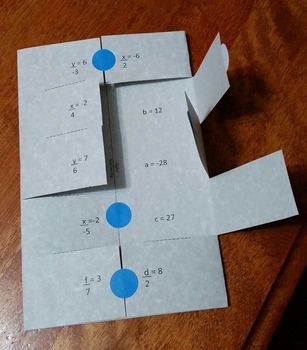Solving One and Two Step Equations "Solve and Flip" Algebra Activity and GameSubject
Resource Type
Product Rating
4.0
5 Ratings
File Type

PDF (Acrobat) Document File

Be sure that you have an application to open this file type before downloading and/or purchasing.

591 KB|105 pages
Share
Product Description
This download includes 50 different "solve and flip" algebra sheets. There are 10 problems per sheet, for a total of 500 different problems. These sheets can be used as a differentiated independent activity, math game, or an addition to your existing math centers. The different levels of difficulty will enable you to provide extra practice or enrichment for your middle school students.

Included in this download - self-checking Solve and Flip sheets (5 of each, for a total of 50 different sheets):
-One step equations using addition/subtraction to solve
-One step equations using division to solve
-One step equations using division to solve (with negatives)
-One step equations using multiplication to solve
-One step equations using multiplication to solve (with negatives)
-Two step equations using addition/subtraction and division to solve
-Two step equations using addition/subtraction and division to solve (with negatives)
-Two step equations using addition/subtraction and multiplication to solve
-Two step equations using addition/subtraction and multiplication to solve (with negatives)
-Two step equations using the distributive property

-game rules sheet

Teacher-author: Krystal Mills
Total Pages
105 pages
Does not apply
Teaching Duration
N/A
Report this Resource to TpT
Reported resources will be reviewed by our team. Report this resource to let us know if this resource violates TpT’s content guidelines.